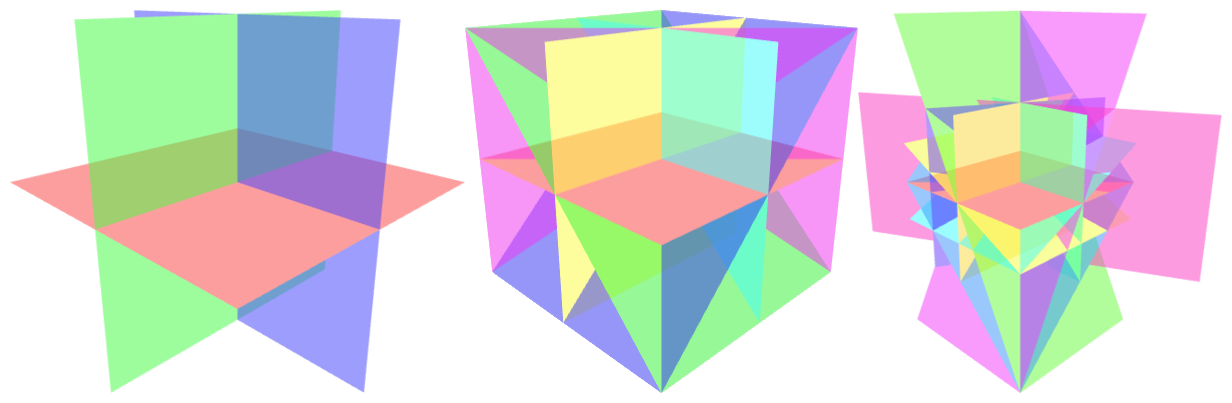# Solving $k$-SUM using few linear queries

### Abstract

The $k$-SUM problem is given $n$ input real numbers to determine whether any $k$ of them sum to zero. The problem is of tremendous importance in the emerging field of complexity theory within $P$, and it is in particular open whether it admits an algorithm of complexity $O(n^c)$ with $c<\lceil \frac{k}{2} \rceil$. Inspired by an algorithm due to Meiser (1993), we show that there exist linear decision trees and algebraic computation trees of depth $O(n^3\log^2 n)$ solving $k$-SUM. Furthermore, we show that there exists a randomized algorithm that runs in $\tilde{O}(n^{\lceil\frac{k}{2}\rceil+8})$ time, and performs $O(n^3\log^2 n)$ linear queries on the input. Thus, we show that it is possible to have an algorithm with a runtime almost identical (up to the $+8$) to the best known algorithm but for the first time also with the number of queries on the input a polynomial that is independent of $k$. The $O(n^3\log^2 n)$ bound on the number of linear queries is also a tighter bound than any known algorithm solving $k$-SUM, even allowing unlimited total time outside of the queries. By simultaneously achieving few queries to the input without significantly sacrificing runtime vis-à-vis known algorithms, we deepen the understanding of this canonical problem which is a cornerstone of complexity-within-$P$. We also consider a range of tradeoffs between the number of terms involved in the queries and the depth of the decision tree. In particular, we prove that there exist $o(n)$-linear decision trees of depth $\tilde{O}(n^3)$ for the $k$-SUM problem.

Publication
In European Symposium on Algorithms (ESA 2016)